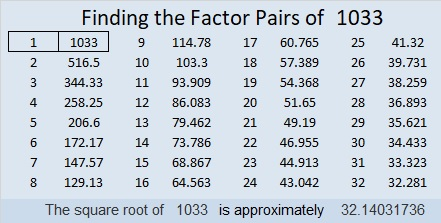# 1033 and Level 3

To solve a level 3 puzzle begin with 80, the clue at the very top of the puzzle. Clue 48 goes with it. What are the factor pairs of those numbers in which both factors are between 1 and 12 inclusive? 80 can be 8×10, and 48 can be 4×12 or 6×8. What is the only number that listed for both 80 and 48? Put that number in the top row over the 80. Put the corresponding factors where they go starting at the top of the first column.

Work down that first column cell by cell finding factors and writing them as you go. Three of the factors have been highlighted because you have to at least look at the 55 and the 5 to deal with the 20 in the puzzle. Have fun!Print the puzzles or type the solution in this excel file: 12 factors 1028-1034

Here are a few facts about the number 1033:

It is a twin prime with 1031.

32² + 3² = 1033, so it is the hypotenuse of a Pythagorean triple:
192-1015-1033 calculated from 2(32)(3), 32² – 3², 32² + 3²

1033 is a palindrome in two other bases:
It’s 616 in BASE 13 because 6(13²) + 1(13) + 6(1) = 1033
1J1 in BASE 24 (J is 19 base 10) because 24² + 19(24) + 1 = 1033

8¹ + 8º + 8³ + 8³ = 1033 Thanks to OEIS.org for that fun fact!

• 1033 is a prime number.
• Prime factorization: 1033 is prime.
• The exponent of prime number 1033 is 1. Adding 1 to that exponent we get (1 + 1) = 2. Therefore 1033 has exactly 2 factors.
• Factors of 1033: 1, 1033
• Factor pairs: 1033 = 1 × 1033
• 1033 has no square factors that allow its square root to be simplified. √1033 ≈ 32.1403

How do we know that 1033 is a prime number? If 1033 were not a prime number, then it would be divisible by at least one prime number less than or equal to √1033 ≈ 32.1. Since 1033 cannot be divided evenly by 2, 3, 5, 7, 11, 13, 17, 19, 23, 29 or 31, we know that 1033 is a prime number.Here’s another way we know that 1033 is a prime number: Since its last two digits divided by 4 leave a remainder of 1, and 32² + 3² = 1033 with 32 and 3 having no common prime factors, 1033 will be prime unless it is divisible by a prime number Pythagorean triple hypotenuse less than or equal to √1033 ≈ 32.1. Since 1033 is not divisible by 5, 13, 17, or 29, we know that 1033 is a prime number.

This site uses Akismet to reduce spam. Learn how your comment data is processed.